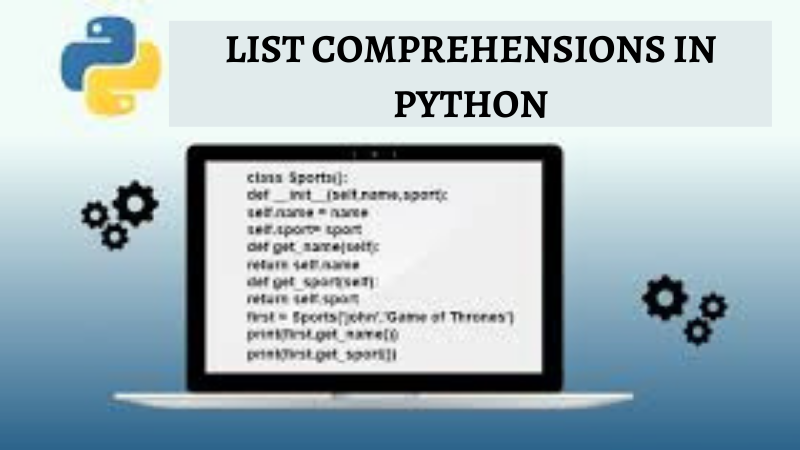/  Technology   /  LIST COMPREHENSIONS IN PYTHON## LIST COMPREHENSIONS IN PYTHON

#### Quick Recap of Lists

Lists belong to the four built-in data structures in Python. It’s a compound(or)heterogeneous data type i.e., the elements need not be of the same type: they can be a combination of Boolean, string, integer, float values.

Note: Lists are ordered collections of items or objects. This makes lists act as sequences. Strings, tuples or sets are also examples of sequences.

Accessing Elements from a List can be done using Indexing and Slicing.

```>>list= [0, 2, 4, 6, 8, 10]
>>print(list)
4
>>print(list[:3])
[0,2,4]```

Now that we quickly revised about lists in brief, let’s jump into the main topic. Python is known for its easy, readable and user-friendly code. As we have seen for entering elements into the list, the developer has to either append each element or use a for loop. This is quite a tedious task to do, even using a for loop can make the code lengthy. Let’s see an example.

#### #using For loop:

```>>> l = []
>>> for x in range (6):
l.append(x**2)
>>> l
[0, 1, 4, 9, 16, 25]```

We should take advantage of Python’s special feature “List Comprehension” which will turn the above-mentioned lengthy code to

```>>> [x**2 for x in range (6)]
[0, 1, 4, 9, 16, 25]```

List comprehension in Python is also bounded within square brackets, but instead of the list of data inside it, we enter an expression followed by for loops and if-else clauses.

Syntax:

`list_variable = [expression for iterator in sequence]`

The above syntax if you can relate, closely resembles the set builder notation in mathematics.

Consider this example:

`S = {x² : x in {0 ... 5}}`

S is a sequence that contains values between 0 and 5 included, and each value is raised to the power of two. So, the set of S looks like:

`S = {0, 1, 4, 9, 16, 25}`

Now let’s relate this theory to our list comprehension:

`>>S = [x**2 for x in range(6)]`

Let’s break down this piece of code

• EXPRESSION is x ** 2
• VARIABLE(or) ITERATOR  is x
• SEQUENCE is range(6)

The output would be S=[0, 1, 4, 9, 25]

The Expression can be anything that concludes to a value:

•       An arithmetic expression such as n ** 5 / 3 * n + 7.
•       A function call similar to f(n), using n as a variable.
•       A slice operation like T[2::-1]  etc.

If we only want to extract expression output for a specific set of data from the entire collection of elements, we can use an if clause after the for loop. The expression will be added to the list only if the if clause is ‘True’. Even more than one if clause is allowed, and only if the if clauses return True, do the expressions stay in the list.

```>>M = [x for x in S if x % 2 == 0]
[0, 4, 16]```

While using the if-else clause ternary expression, we need to put the if… else..after x, not after range(6).So the syntax would be:

Syntax:

`variable if expression else expression for variable sequence`
``` >> i if i%2 != 0 else even for i in range(6)]
[even, 1, even, 3, even, 5]```

Reference

List comprehension